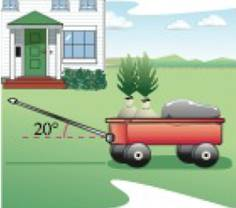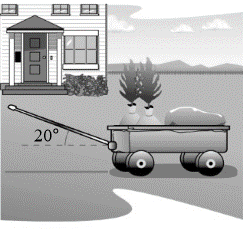Chapter 11.3, Problem 62E

Chapter
Section
Textbook Problem

# WorkA wagon is pulled by exerting a force of 65 pounds on a handle that makes a 20° angle with the horizontal (see figure). Find the work done in pulling the wagon 50 feet.To determine

To calculate: The amount of the work done in pulling the wagon 50 feet when a force of 65 pounds

acts on the wagon at the handle which makes an angle of 200 to the horizontal.Explanation

Given:

A force of 65 pounds acts on the wagon at the handle which makes an angle of 200 to the horizontal.

Formula used:

Work done when a force of F displaces an object by the displacement s is given by W=Fs.

Calculation:

The force vector is given as, F=65[cos(20°)i+sin(20°)j].

The displacement vector can be written as;

s=50i

### Still sussing out bartleby?

Check out a sample textbook solution.

See a sample solution

#### The Solution to Your Study Problems

Bartleby provides explanations to thousands of textbook problems written by our experts, many with advanced degrees!

Get Started